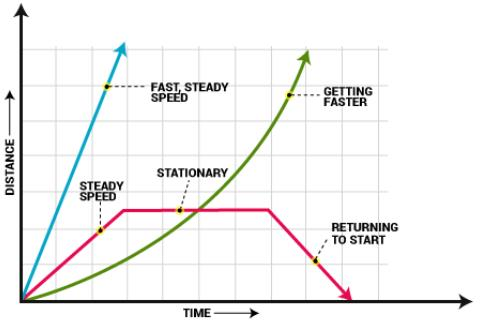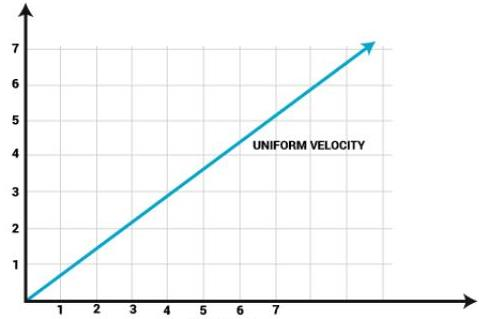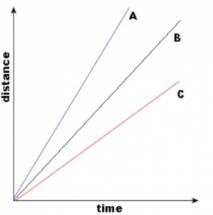Courses

# Test: Distance Time Graphs

## 5 Questions MCQ Test Science Class 9 | Test: Distance Time Graphs

Description
This mock test of Test: Distance Time Graphs for Class 9 helps you for every Class 9 entrance exam. This contains 5 Multiple Choice Questions for Class 9 Test: Distance Time Graphs (mcq) to study with solutions a complete question bank. The solved questions answers in this Test: Distance Time Graphs quiz give you a good mix of easy questions and tough questions. Class 9 students definitely take this Test: Distance Time Graphs exercise for a better result in the exam. You can find other Test: Distance Time Graphs extra questions, long questions & short questions for Class 9 on EduRev as well by searching above.
QUESTION: 1

### In a distance-time graph, if the line is horizontal, then the object is:Solution:

When an object is at rest it will not be able to cover any distance . So for any object at rest the distance -time graph is horizontal to time axis

QUESTION: 2

### On the distance-time graph, the Y-axis should be labeled as:Solution:

Independent variables are plotted along X-axis and Dependent variables are plotted along Y-axis. In the distance-time graph, time is independent and distance is a dependent variables.

QUESTION: 3

### The slope of the distance-time graph is:

Solution:
The gradient of a distance-time graph represents the speed of an object. The velocity of an object is its speed in a particular direction. The slope on a velocity-time graph represents the acceleration of an object. The distance travelled is equal to the area under a velocity-time graph.

QUESTION: 4

For a body performing motion with uniform speed, the distance-time graph is:

Solution:

For a body moving at uniform speed, the distance time graph is a straight line inclined to the time axis, which may pass through origin or some other point on the y- axis.As in uniform motion, the distance time graph would be a straight line, because the equal distance is covered in equal units of time.

QUESTION: 5

What is the slope of distance-time graph when object is at rest?

Solution: Since The Body is at Rest ,It clearly means that The Body hasn't covered any Distance here.So,Distance is equal to zero.So,There is no meaning of having any slope here.. So,The Slope=0 Becoz The Distance at anytime will represent same point in Distance-Time Graph at 0..So,(B)is d correct answer. .